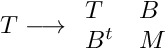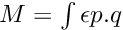GetFEM++  5.3
Finite element description

## Classes

class  getfem::virtual_fem
Base class for finite element description. More...

class  getfem::fem< FUNC >
virtual_fem implementation as a vector of generic functions. More...

class  getfem::fem_precomp_
Pre-computations on a fem (given a fixed set of points on the reference convex, this object computes the value/gradient/hessian of all base functions on this set of points and stores them. More...

class  getfem::fem_precomp_pool
handle a pool (i.e. More...

class  getfem::fem_interpolation_context
structure passed as the argument of fem interpolation functions. More...

## Typedefs

typedef std::shared_ptr< const getfem::virtual_femgetfem::pfem
type of pointer on a fem description More...

typedef const fem< bgeot::base_poly > * getfem::ppolyfem
Classical polynomial FEM. More...

typedef const fem< bgeot::polynomial_composite > * getfem::ppolycompfem
Polynomial composite FEM.

typedef const fem< bgeot::base_rational_fraction > * getfem::prationalfracfem
Rational fration FEM.

## Functions

pfem getfem::classical_fem (bgeot::pgeometric_trans pgt, short_type k, bool complete=false)
Give a pointer on the structures describing the classical polynomial fem of degree k on a given convex type. More...

pfem getfem::classical_discontinuous_fem (bgeot::pgeometric_trans pg, short_type k, scalar_type alpha=0, bool complete=false)
Give a pointer on the structures describing the classical polynomial discontinuous fem of degree k on a given convex type. More...

pfem getfem::fem_descriptor (const std::string &name)
get a fem descriptor from its string name. More...

std::string getfem::name_of_fem (pfem p)
get the string name of a fem descriptor. More...

pfem_precomp getfem::fem_precomp (pfem pf, bgeot::pstored_point_tab pspt, dal::pstatic_stored_object dep)
Handles precomputations for FEM. More...

void getfem::delete_fem_precomp (pfem_precomp pfp)
Request for the removal of a pfem_precomp.

template<typename CVEC , typename VVEC >
void getfem::virtual_fem::interpolation (const fem_interpolation_context &c, const CVEC &coeff, VVEC &val, dim_type Qdim) const
Interpolate at an arbitrary point x given on the reference element. More...

template<typename MAT >
void getfem::virtual_fem::interpolation (const fem_interpolation_context &c, MAT &M, dim_type Qdim) const
Build the interpolation matrix for the interpolation at a fixed point x, given on the reference element. More...

template<typename CVEC , typename VMAT >
void getfem::virtual_fem::interpolation_grad (const fem_interpolation_context &c, const CVEC &coeff, VMAT &val, dim_type Qdim=1) const

template<typename CVEC , typename VMAT >
void getfem::virtual_fem::interpolation_hess (const fem_interpolation_context &c, const CVEC &coeff, VMAT &val, dim_type Qdim) const
Interpolation of the hessian. More...

template<typename CVEC >
void getfem::virtual_fem::interpolation_diverg (const fem_interpolation_context &c, const CVEC &coeff, typename gmm::linalg_traits< CVEC >::value_type &val) const
Interpolation of the divergence. More...

## Typedef Documentation

 typedef std::shared_ptr getfem::pfem

type of pointer on a fem description

getfem::virtual_fem

Definition at line 239 of file getfem_fem.h.

 typedef const fem* getfem::ppolyfem

Classical polynomial FEM.

Definition at line 594 of file getfem_fem.h.

## Function Documentation

 pfem getfem::classical_fem ( bgeot::pgeometric_trans pgt, short_type k, bool complete = false )

Give a pointer on the structures describing the classical polynomial fem of degree k on a given convex type.

Parameters
 pgt the geometric transformation (which defines the convex type). k the degree of the fem. complete a flag which requests complete Langrange polynomial elements even if the provided pgt is an incomplete one (e.g. 8-node quadrilateral or 20-node hexahedral).
Returns
a ppolyfem.

Definition at line 3867 of file getfem_fem.cc.

 pfem getfem::classical_discontinuous_fem ( bgeot::pgeometric_trans pg, short_type k, scalar_type alpha = 0, bool complete = false )

Give a pointer on the structures describing the classical polynomial discontinuous fem of degree k on a given convex type.

Parameters
 pgt the geometric transformation (which defines the convex type). k the degree of the fem. alpha the "inset" factor for the dof nodes: with alpha = 0, the nodes are located as usual (i.e. with node on the convex border), and for 0 < alpha < 1, they converge to the center of gravity of the convex. complete a flag which requests complete Langrange polynomial elements even if the provided pgt is an incomplete one (e.g. 8-node quadrilateral or 20-node hexahedral).
Returns
a ppolyfem.

Definition at line 3872 of file getfem_fem.cc.

 pfem getfem::fem_descriptor ( const std::string & name )

get a fem descriptor from its string name.

Definition at line 3950 of file getfem_fem.cc.

 std::string getfem::name_of_fem ( pfem p )

get the string name of a fem descriptor.

Definition at line 3959 of file getfem_fem.cc.

 pfem_precomp getfem::fem_precomp ( pfem pf, bgeot::pstored_point_tab pspt, dal::pstatic_stored_object dep )

Handles precomputations for FEM.

statically allocates a fem-precomputation object, and returns a pointer to it. The fem_precomp_ objects are "cached", i.e. they are stored in a global pool and if this function is called two times with the same arguments, a pointer to the same object will be returned.

Parameters
 pf a pointer to the fem object. pspt a pointer to a list of points in the reference convex.CAUTION: this array must not be destroyed as long as the fem_precomp is used!!.

Moreover pspt is supposed to identify uniquely the set of points. This means that you should NOT alter its content at any time after using this function.

If you need a set of "temporary" getfem::fem_precomp_, create them via a getfem::fem_precomp_pool structure. All memory will be freed when this structure will be destroyed.

Definition at line 4051 of file getfem_fem.cc.

template<typename CVEC , typename VVEC >
 void getfem::virtual_fem::interpolation ( const fem_interpolation_context & c, const CVEC & coeff, VVEC & val, dim_type Qdim ) const

Interpolate at an arbitrary point x given on the reference element.

Parameters
 c the fem_interpolation_context, should have been suitably initialized for the point of evaluation. coeff is the vector of coefficient relatively to the base functions, its length should be Qdim*this->nb_dof(). val contains the interpolated value, on output (its size should be Qdim*this->target_dim()). Qdim is the optional Q dimension, if the FEM is considered as a "vectorized" one.

Definition at line 850 of file getfem_fem.h.

template<typename MAT >
 void getfem::virtual_fem::interpolation ( const fem_interpolation_context & c, MAT & M, dim_type Qdim ) const

Build the interpolation matrix for the interpolation at a fixed point x, given on the reference element.

The matrix M is filled, such that for a given coeff vector, the interpolation is given by M*coeff.

Definition at line 872 of file getfem_fem.h.

template<typename CVEC , typename VMAT >
 void getfem::virtual_fem::interpolation_grad ( const fem_interpolation_context & c, const CVEC & coeff, VMAT & val, dim_type Qdim = 1 ) const

The output is stored in thematrix val.

Definition at line 894 of file getfem_fem.h.

template<typename CVEC , typename VMAT >
 void getfem::virtual_fem::interpolation_hess ( const fem_interpolation_context & c, const CVEC & coeff, VMAT & val, dim_type Qdim ) const

Interpolation of the hessian.

The output is stored in thematrix val.

Definition at line 921 of file getfem_fem.h.

template<typename CVEC >
 void getfem::virtual_fem::interpolation_diverg ( const fem_interpolation_context & c, const CVEC & coeff, typename gmm::linalg_traits< CVEC >::value_type & val ) const

Interpolation of the divergence.

The output is stored in the scalar val.

Definition at line 949 of file getfem_fem.h.